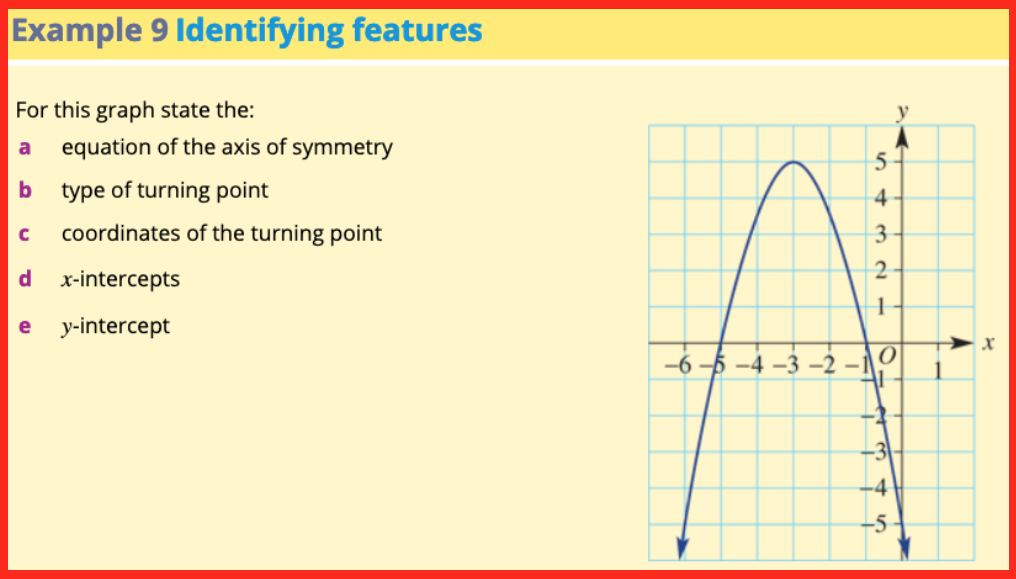# WitCH 14: Stretching the Truth

The easy WitCH below comes courtesy of the Evil Mathologer. It is a worked example from Cambridge’s Essential Mathematics Year 9 (2019), in a section introducing parabolic graphs.## Update

The problem, as commenters have indicated below, is that there is no parabola with the indicated turning point and intercepts. Normally, we’d write this off as a funny but meaningless error. But, coming at the very beginning of the introduction to the parabola, it most definitely qualifies as crap.

## 4 Replies to “WitCH 14: Stretching the Truth”

1.Red Five says:

There is no quadratic function which can have a vertex and axial intercepts where pictured.

If we ignore the x-intercepts, the graph has form y=a(x+3)^2 +5. Using (0,-5) gives a=-10/9

If, on the other hand we ignore the turning point the graph has form y=a(x+1)(x+5). Using (0,-5) gives a=-1.

Which is close, but not the same as -10/9.

Have I missed anything?

1.marty says:

Yep, that’s it. It’s simply not a parabola.

2.Red Five says:

It’s not even a picture of a parabola…

3.Alasdair says:

What a hoot. To fit the three intercepts and the maximum (-3,5) it would have to be a cubic function, whose graph is not symmetrical. To be symmetrical – so that the graph passes through (-6,-5) – it would have to be a quartic function. My guess is that the displayed graph is the latter, but then of course no part of the graph of a quartic function is a parabola.